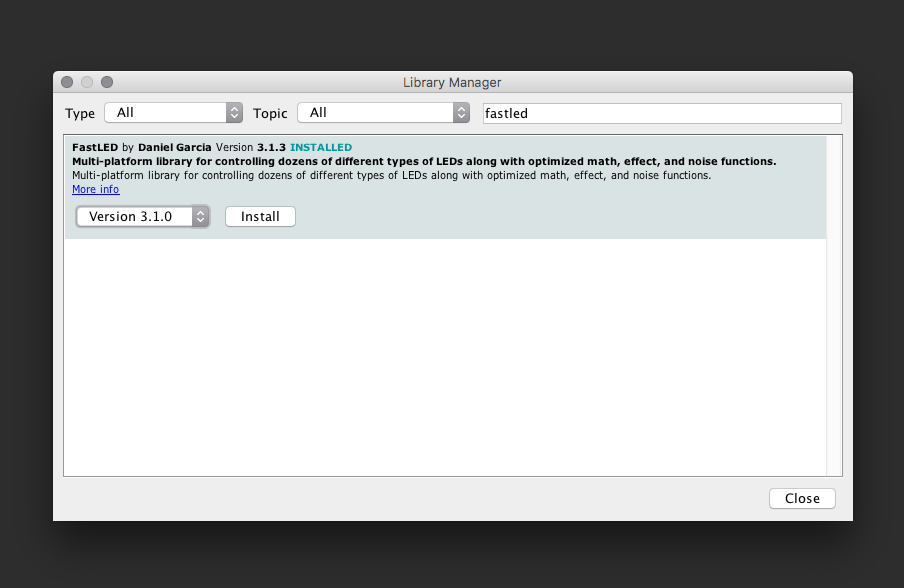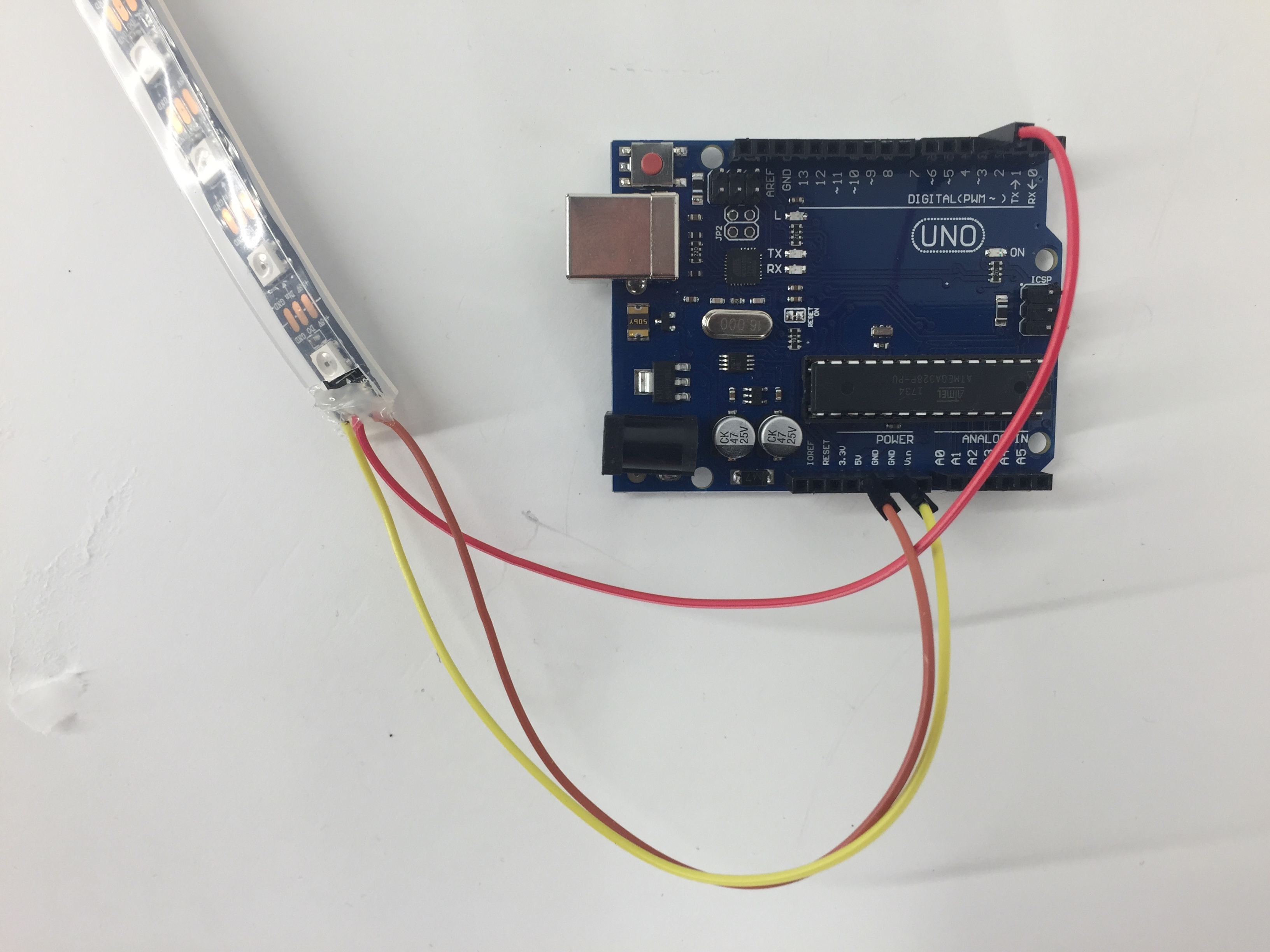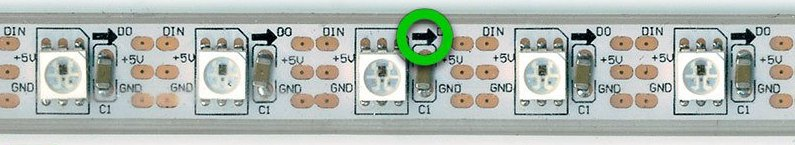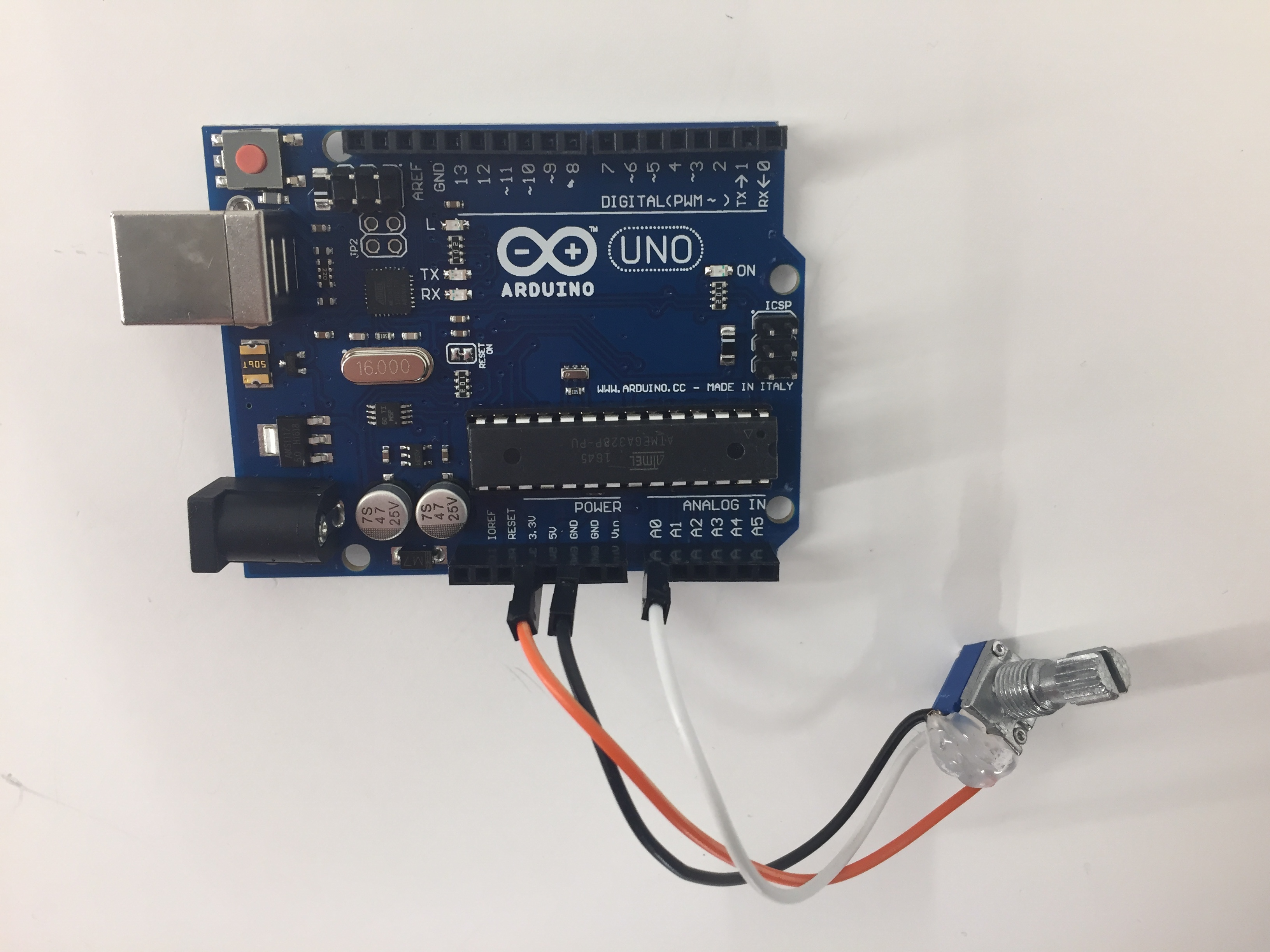Published: August 27, 2018, Edited by: Sara Almeida Santos Daugbjerg

# Introduction to Arduino

Arduino is an open-source electronics platform that is used to sense the real world, make interactive projects and prototypes.

The Arduino board is able to read inputs and write outputs. In this example, the input will be a potentiometer (a variable resistor) and the output a string of neo-pixels - individually controllable light emitting diodes. Using code, we can control how the input affects the output.

Download the Arduino software here: Arduino. This is used to program the Arduino microcontroller. Thereafter, the program is in the Arduino, and will continue to run without the computer present.

## Control neopixels

Neopixels are versatile light strips where each diode can be controlled individually. Each diode or pixel has red, green, and blue - each can be from 0 to 255 in brightness. Multiple libraries exist to more easily control neopixels. We suggest you use "Fastled".

Add the library: "menu->sketch->include library->manage libraries"Search for "fastled" and click installUse this wiring:IMPORTANT: Disconnect the Arduino from power, and connectthe wire labelled 5v on the Neopixel string to 5V on the Arduino, D0 (neopixel end) to digital Pin 4 (Ardunio end) and G (neopixel end) to GND (Arduino end). If you are soldering your own, make sure to connect to the neopixel Din, not Dout - see the arrow:Note the wires we have soldered on are not always the same colours.

This example sketch will turn the first three pixels red, green and blue:

``````  #include "FastLED.h"

// How many leds in your strip?
#define NUM_LEDS 10
#define DATA_PIN 4

// Define the array of leds
CRGB leds[NUM_LEDS];

void setup() {
FastLED.addLeds<NEOPIXEL, DATA_PIN>(leds, NUM_LEDS);
}

void loop() {
// Turn the LED on, then pause
leds= CRGB( 255, 0, 0);
leds= CRGB( 0, 255, 0);
leds= CRGB( 0, 0, 255);
FastLED.show();

}
``````

Exercise: #1 Try to change colors and turn more lights on.

## Adding an input - potentiometer

A potentiometer is a "volume knob" - a variable resistance, which we can read into the Arduino board as an analog value (read more here)

Use this wiring:Left pin = black wire to GND on Arduino,

Middle pin = white or yellow wire to A0 on Arduino

Right pin = red wire to 3.3V on Arduino.

(We only use the 3.3V pin as we have already used the 5V pin for the neopixels - if we had a breadboard or circuit board involved it would be normal to split the 5V and power both with 5V).

In the following code we will let the potentiometer control the brightness of the first pixel.

``````#include "FastLED.h"

// How many leds in your strip?
#define NUM_LEDS 10
#define DATA_PIN 4

// Select the input pin for the potentiometer
int potPin = A0;

// Variable to store the value coming from the sensor
int val;
int bright;

// Define the array of leds
CRGB leds[NUM_LEDS];

void setup() {
FastLED.addLeds<NEOPIXEL, DATA_PIN>(leds, NUM_LEDS);
}

void loop() {

val = analogRead(potPin);
bright = map(val, 0,684,0,255);

leds = CRGB( bright, 0, 0);
leds = CRGB( 0, 255, 0);
leds = CRGB( 0, 0, 255);
FastLED.show();

}
``````

Now we have defined the input pin and two variables. The `int val` will hold the values returned by `analogRead()`. This function reads the values coming in from the potentiometer going from 0 to 684 (when connected to 3.3V) and 0 to 1024 when connected to 5V.

`int bright` is used to define the brightness the first pixel. To do so, the function `map()` is used to re-map the range of values from the potentiometer to the range of values (0-684) used to define the brightness of a neo-pixel (0-255):

Exercise #2 Let the input from the potentiometer control more pixels.

### Making a chase

``````#include "FastLED.h"

// How many leds in your strip?
#define NUM_LEDS 10
#define DATA_PIN 4

// Select the input pin for the potentiometer
int potPin = A0;

// Variable to store the value coming from the sensor
int val;
int brigth;
int ledsOn;

// Define the array of leds
CRGB leds[NUM_LEDS];

void setup() {
FastLED.addLeds<NEOPIXEL, DATA_PIN>(leds, NUM_LEDS);
}

void loop() {

val = analogRead(potPin);
//brigth = map(val, 0, 684, 0, 254);
ledsOn = map(val, 0, 684, 0, NUM_LEDS);

//turn all pixels off
for (int i = 0; i < NUM_LEDS; i = i + 1)
{
leds[i] = CRGB( 0, 0, 0);
}

//Turn the pixels on according to input from potentiometer
for (int i = 0; i < ledsOn; i = i + 1)
{
leds[i] = CRGB( 255, 0, 0);
}
FastLED.show();

}
``````

This time we are using two for-loops. The first turns all the pixels off and the next turns on according to the input from the potentiometer. Without the first loop turning all off they would keep turned on when turning the potentiometer down. Note we are using the variable `ledsOn` to define how many times the loop should run.

Exercise #3 Change the code so there only is one pixel on at a time, and which one is on is controlled by the potentiometer.

## Exercise #3

``````#include "FastLED.h"

// How many leds in your strip?
#define NUM_LEDS 10
#define DATA_PIN 4

// Select the input pin for the potentiometer
int potPin = A0;

// Variable to store the value coming from the sensor
int val;
int brigth;
int ledsOn;
int chase;

// Define the array of leds
CRGB leds[NUM_LEDS];

void setup() {
FastLED.addLeds<NEOPIXEL, DATA_PIN>(leds, NUM_LEDS);
}

void loop() {

val = analogRead(potPin);
brigth = map(val, 0, 684, 0, 254);
ledsOn = map(val, 0, 684, 0, NUM_LEDS);
chase = map(val, 0, 684, 0, NUM_LEDS);

Serial.println(val);

//turn all pixels off
for (int i = 0; i < NUM_LEDS; i = i + 1)
{
leds[i] = CRGB( 0, 0, 0);
}

//Turn the pixels on according to input from potentiometer
leds[chase] = CRGB( 255, 0, 0);

FastLED.show();

}
``````